chillimath.com

## Math Activities for Class 12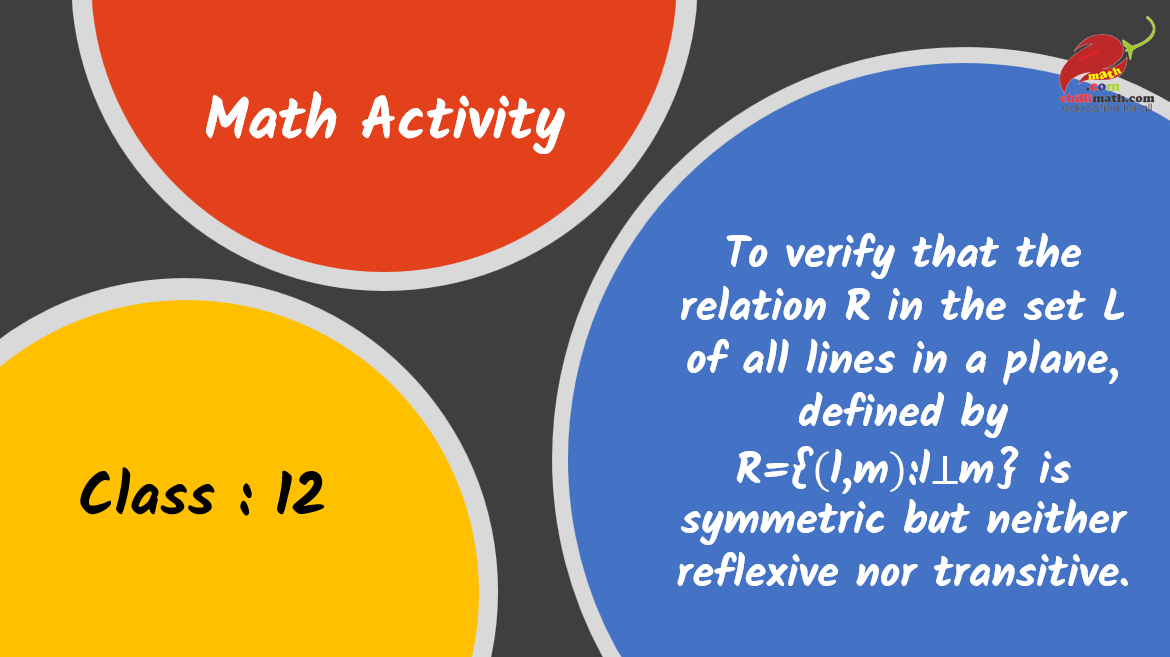Play Video about class 12 math lab activities

#### To explore the principal value of the function $$sin^{−1}⁡𝑥$$ using a unit circle.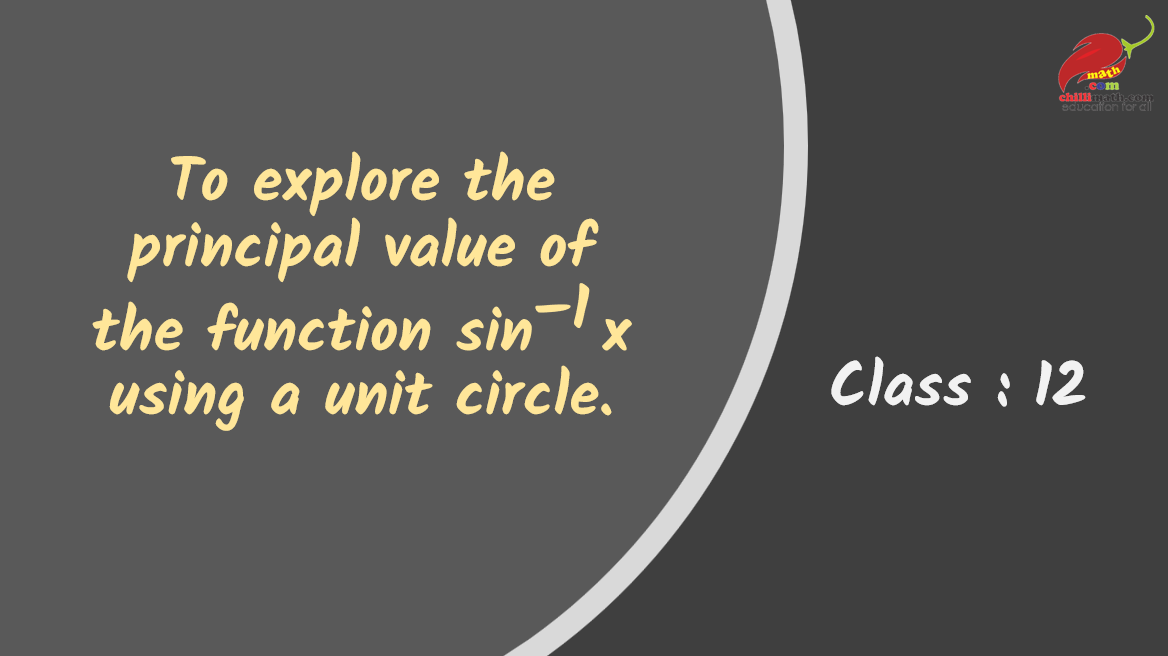Play Video about class 12 math lab activities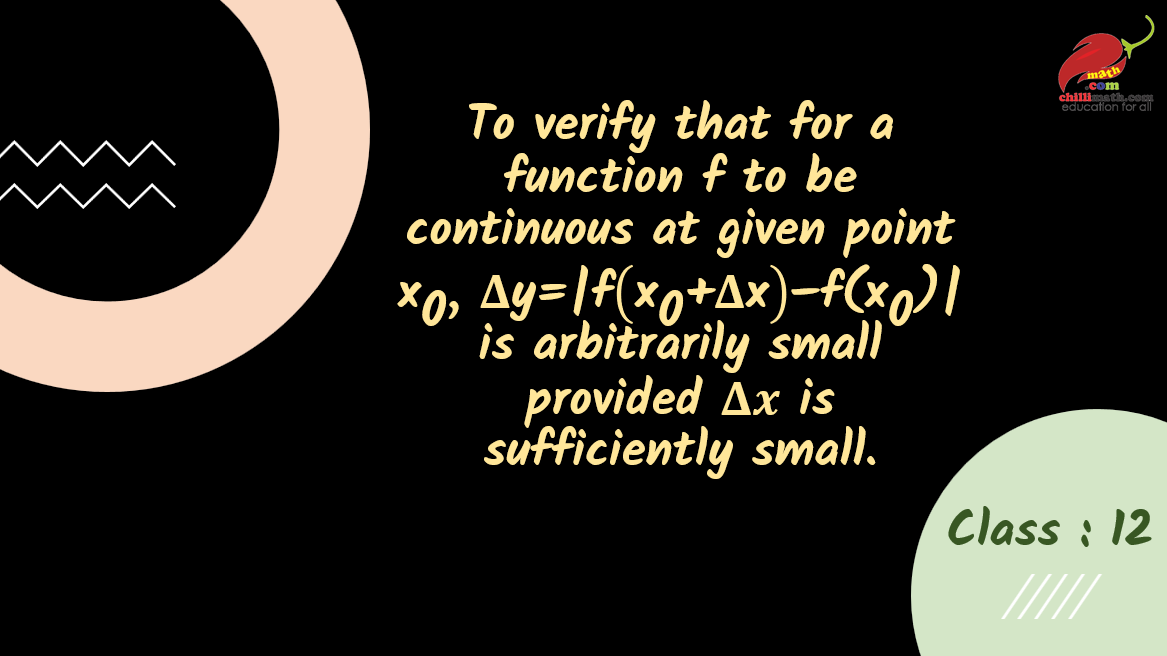Play Video about class 12 math lab activities

#### To understand the concept of local maxima, local minima and point of inflexion.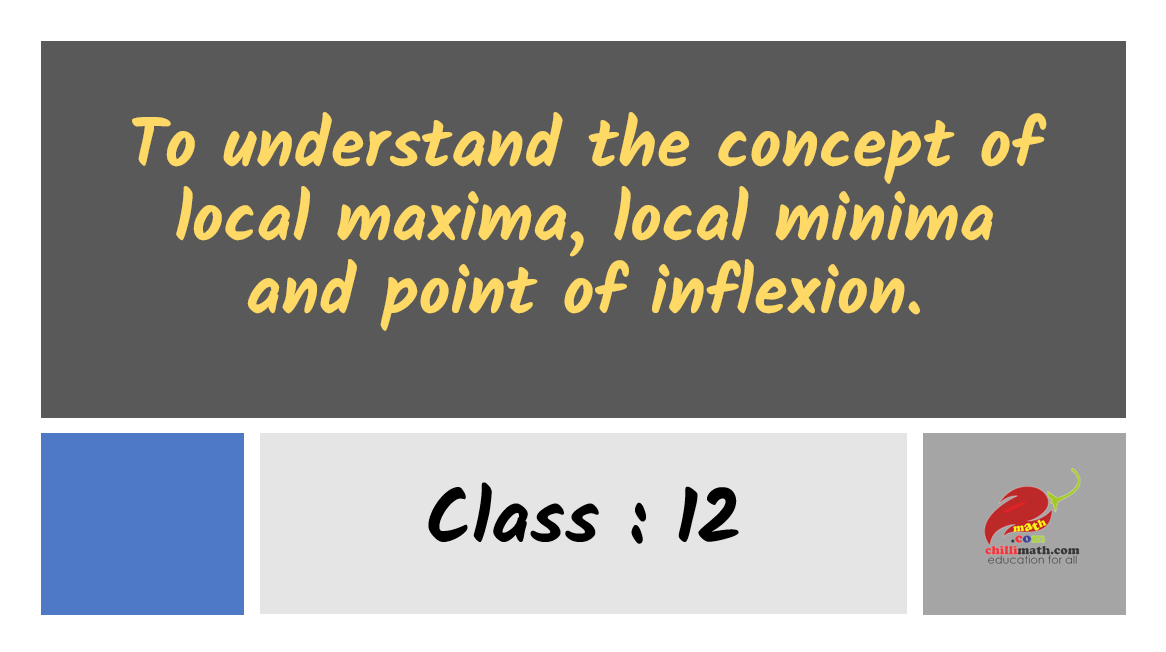Play Video about class 12 math lab activities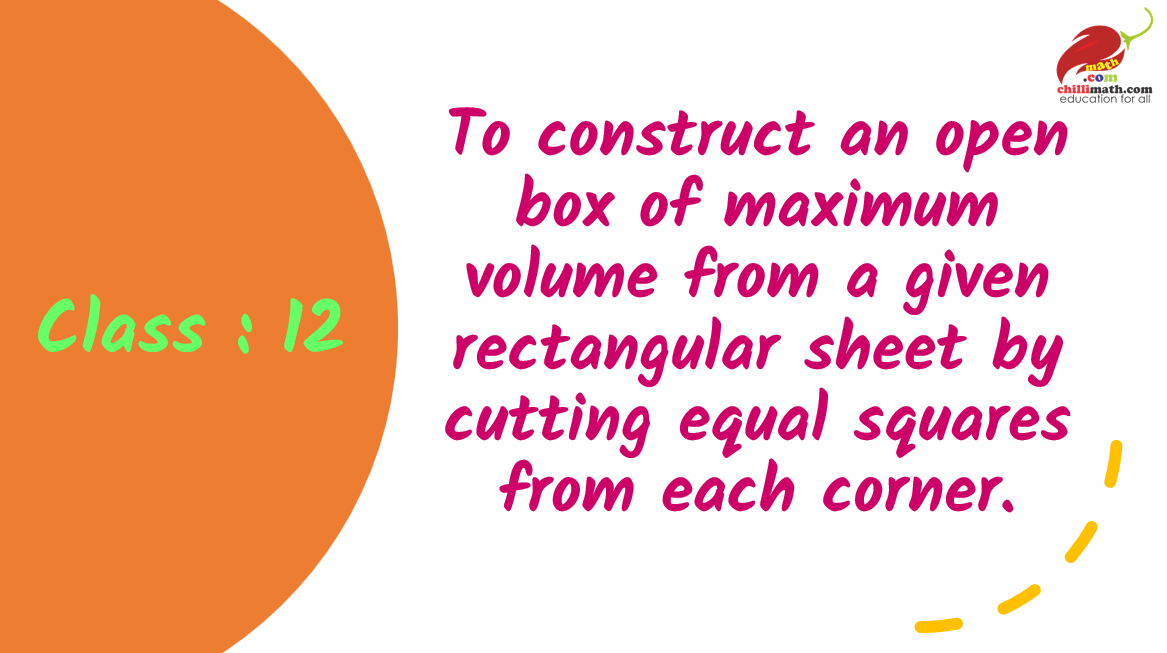Play Video about class 12 math lab activities

## Math Activities for Class 11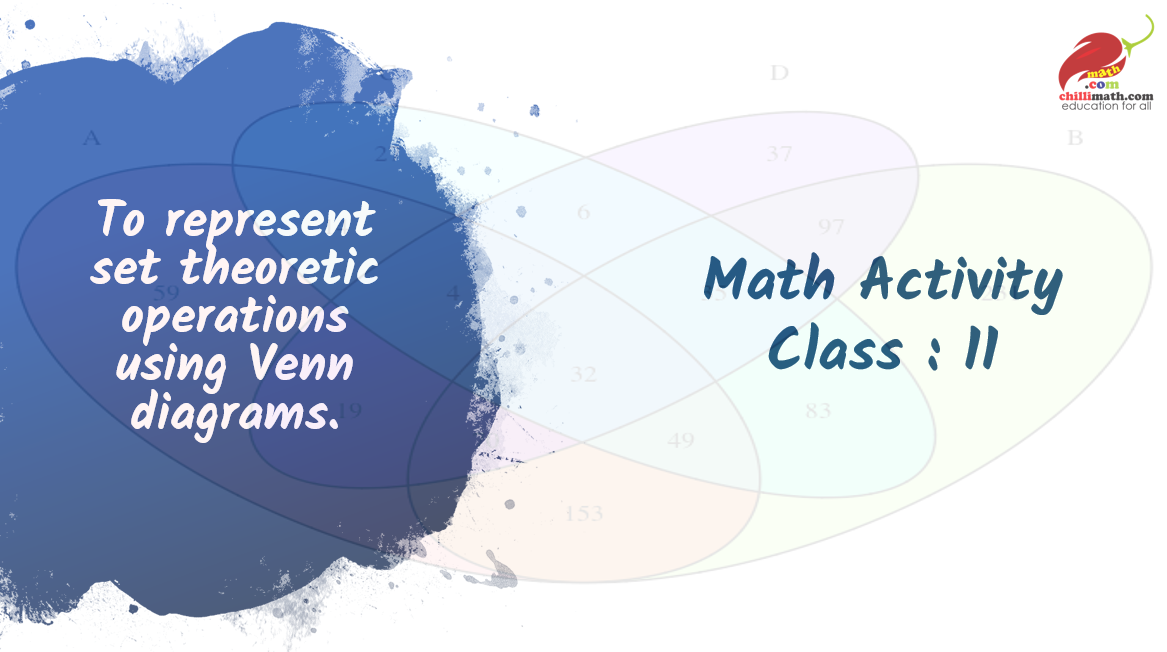Play Video about class 11 math lab activities

#### To find the values of sine and cosine functions in second, third and fourth quadrants using given values in the first quadrant.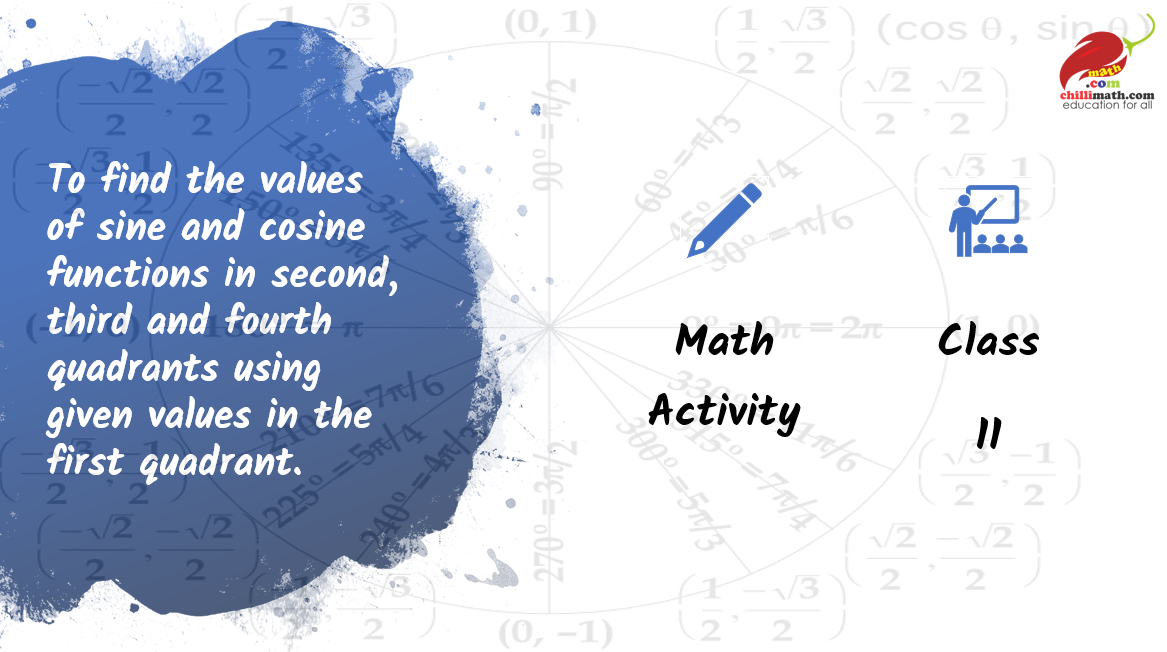Play Video about class 11 math lab activities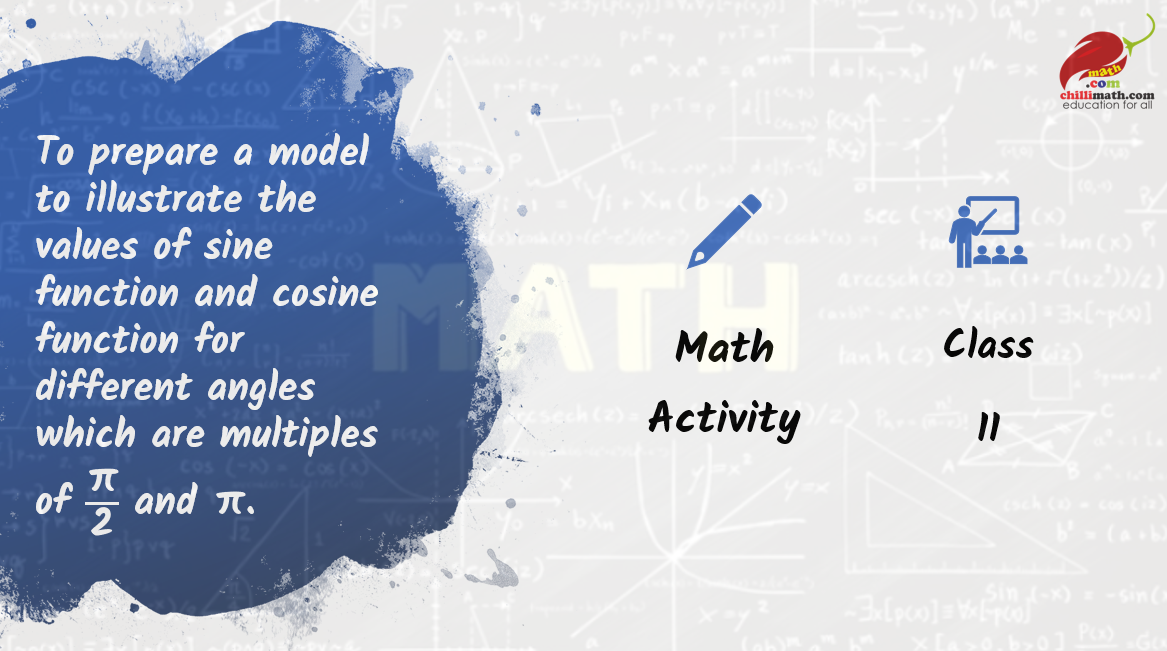Play Video about class 11 math lab activities

#### To interpret geometrically the meaning of “i=” √(“−1” ) and its integral powers.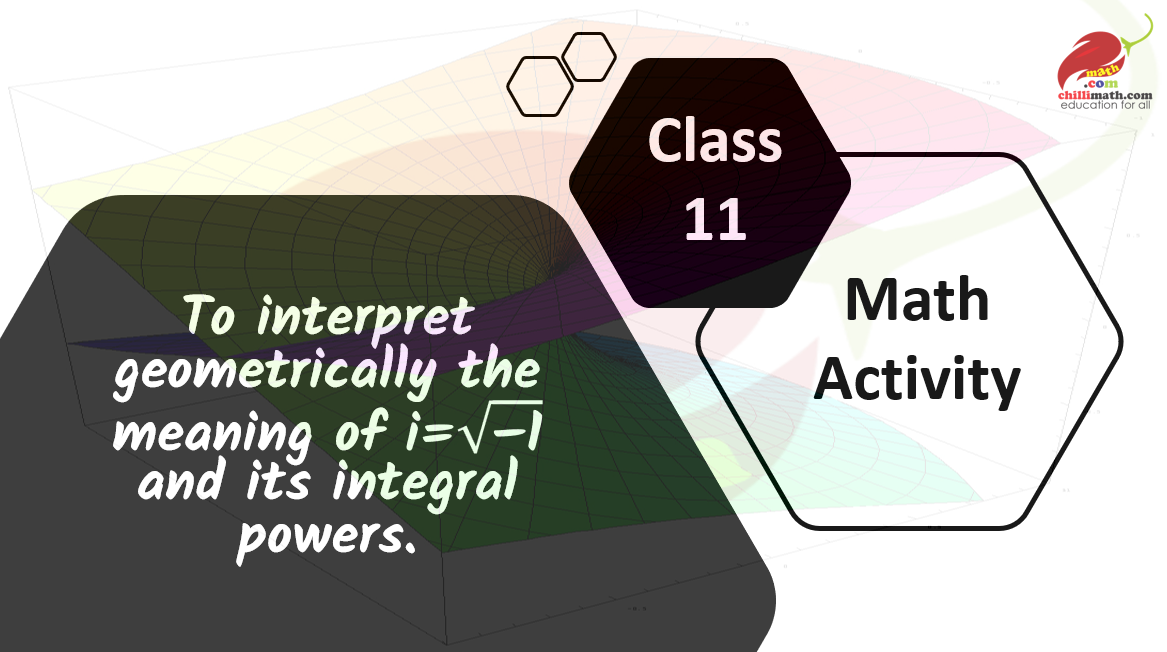Play Video about class 11 math lab activities

## Math Activities for Class 10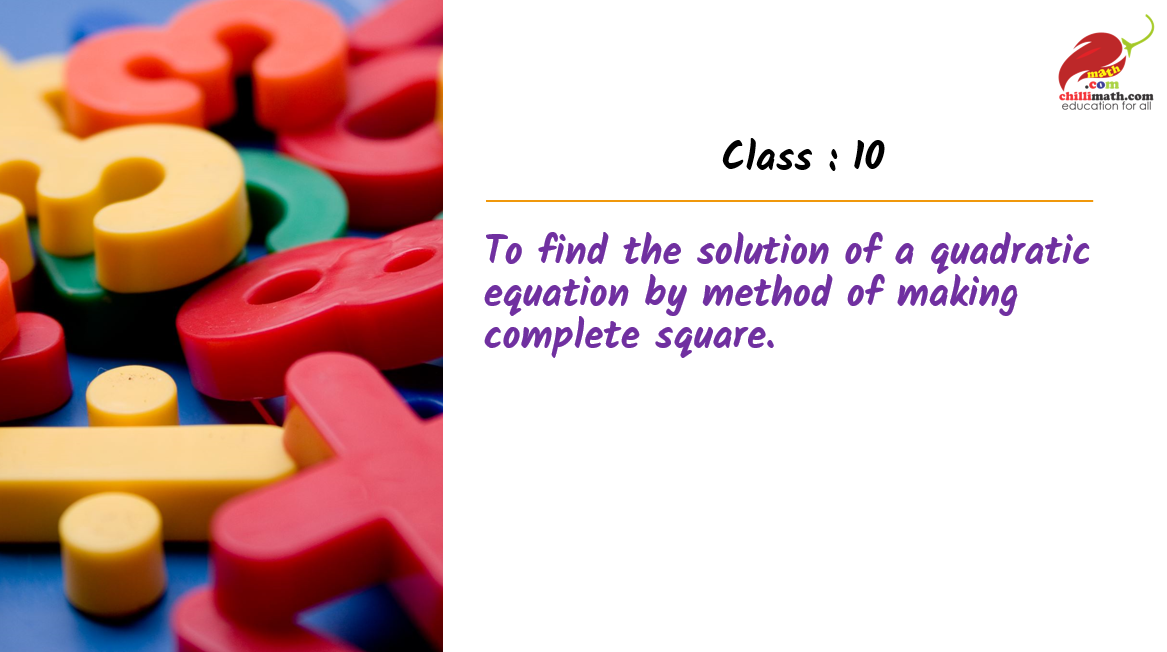Play Video about class 10 math lab activities

#### To verify if a given sequence is an A.P or not by the method of paper cutting and pasting method.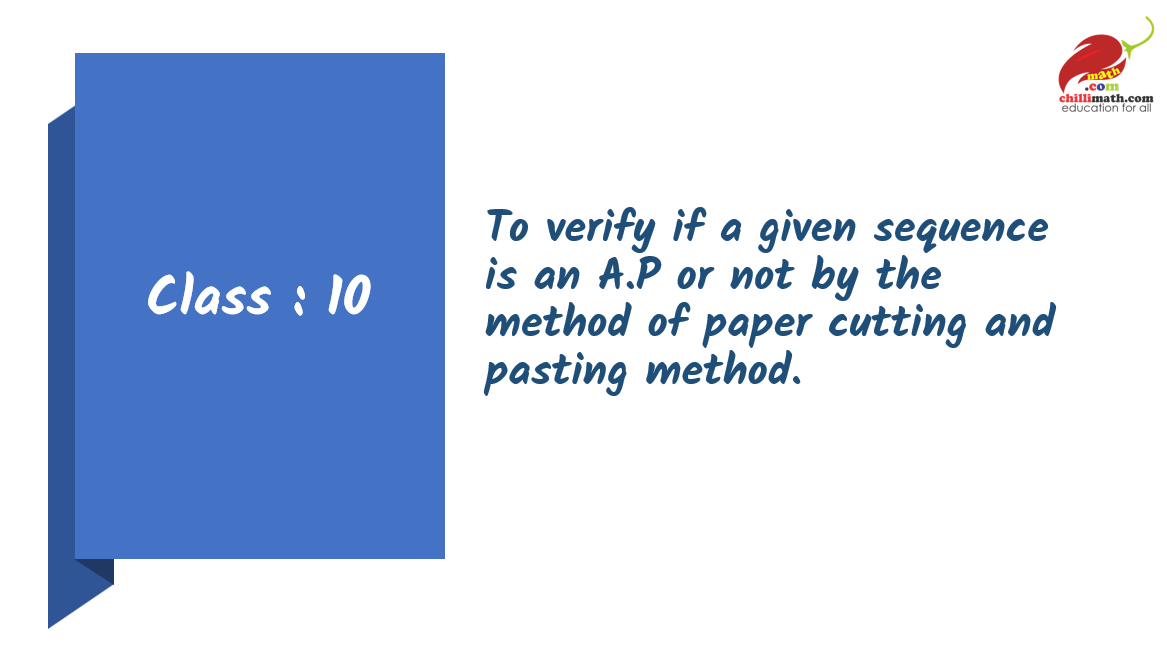Play Video about class 10 math lab activities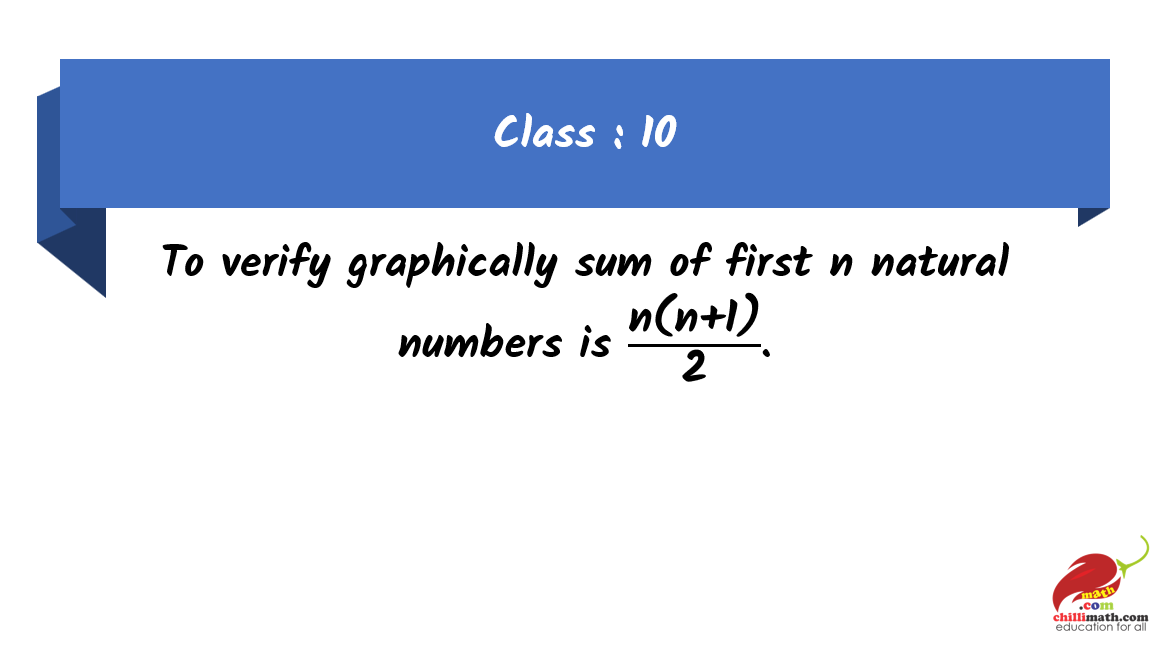Play Video about class 10 math lab activities

#### To obtain the formulae that area of circle is half the product of its circumference and radius.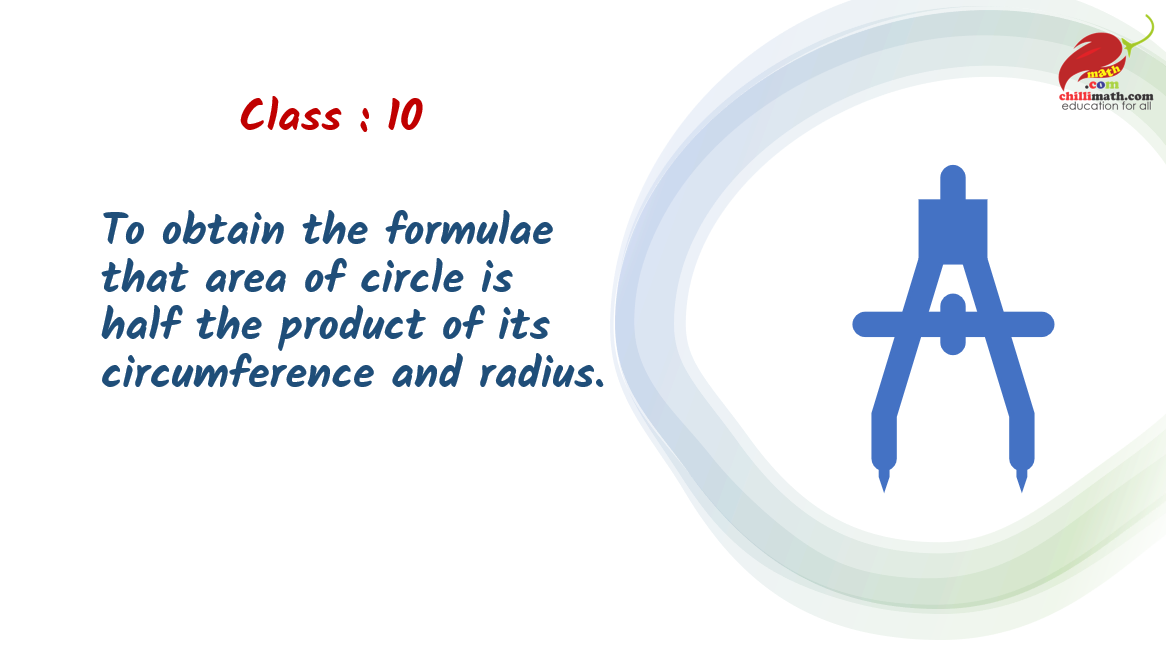Play Video about class 10 math lab activities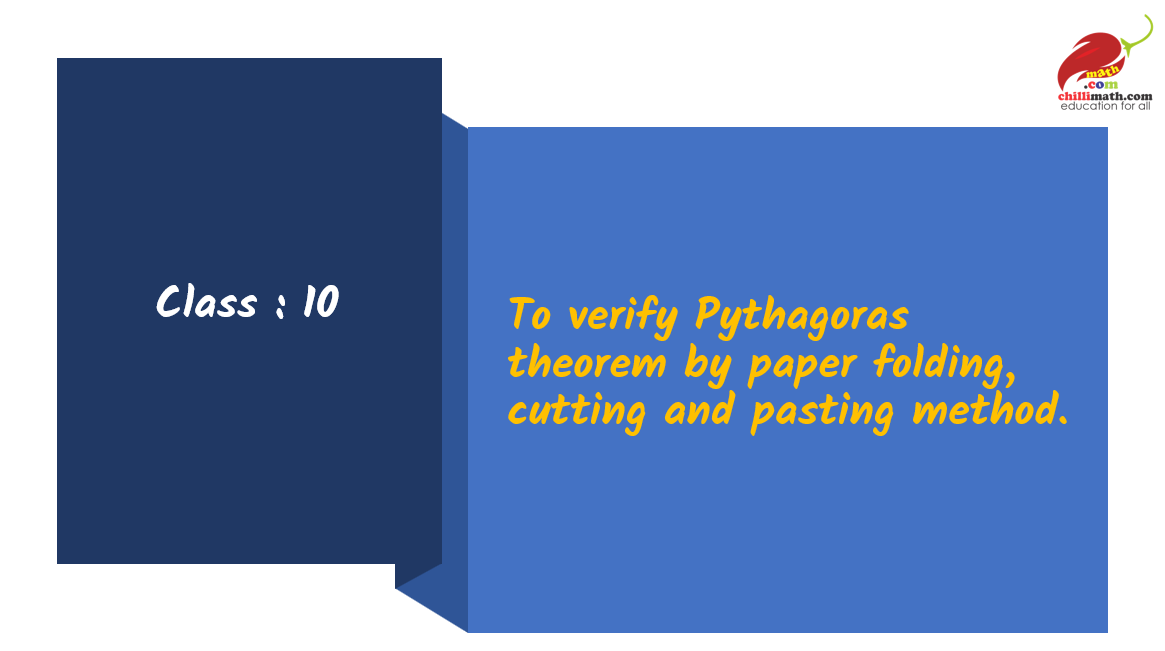Play Video about class 10 math lab activities